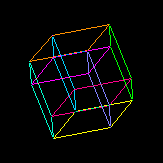# 5-Dim General Relativity (Paul Wesson)Our group is using an extension of general relativity from four to five dimensions where the extra dimension is interpreted as giving rise to matter. Hence the name, Space-Time-Matter theory. Mathematically, STM theory is based on an old embedding theorem of Campbell, which ensures that the 5D Ricci-flat field equations contain the 4D Einstein field equations with matter. Physically, the forms of 4D…